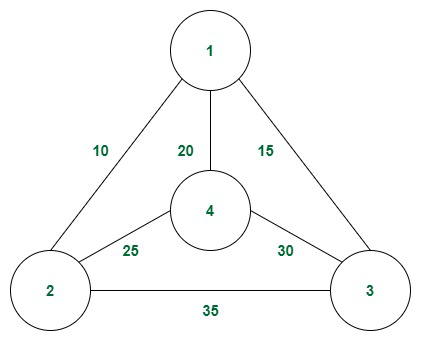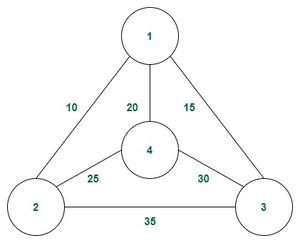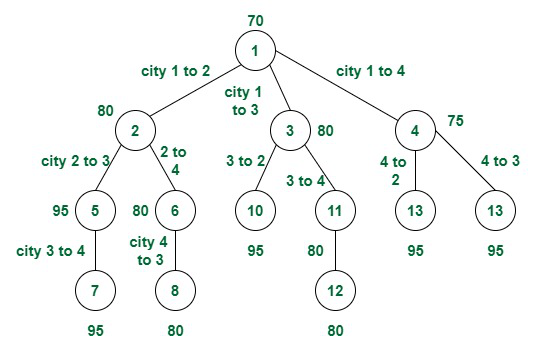Open in App
Not now

# Travelling Salesman Problem (TSP) using Reduced Matrix Method

• Difficulty Level : Hard
• Last Updated : 17 Jun, 2022

Given a set of cities and the distance between every pair of cities, the problem is to find the shortest possible route that visits every city exactly once and returns to the starting point.

Examples:

Input:Example of connections of cities

Output: 80
Explanation: An optimal path is 1 – 2 – 4 – 3 – 1.

Reduced Matrix: This approach is similar to the Branch and Bound approach. The difference here is that the cost of the path and the bound is decided based on the method of matrix reduction. The following are the assumptions for a reduced matrix:

• A row or column of the cost adjacency matrix is said to be reduced if and only if it contains at least one zero element and all remaining entries in that row or column ≥ 0.
• If all rows and columns are reduced then only the matrix is reduced matrix.
• Tour length (new) = Tour length (old) – Total value reduced.
• We first rewrite the original cost adjacency matrix by replacing all diagonal elements from 0 to Infinity

The basic idea behind solving the problem is:

• The cost to reduce the matrix initially is the minimum possible cost for the travelling salesman problem.
• Now in each step, we need to decide the minimum possible cost if that path is taken i.e., a path from vertex u to v is followed.
• We can do that by replacing uth row and vth column cost by infinity and then further reducing the matrix and adding the extra cost for reduction and cost of edge (u, v) with the already calculated minimum path cost.
• Once at least one path cost is found, that is then used as upper bound of cost to apply the branch and bound method on the other paths and the upper bound is updated accordingly when a path with lower cost is found.

Following are the steps to implement the above procedure:

1. Step1: Create a class (Node) that can store the reduced matrix, cost, current city number, level (number of cities visited so far), and path visited till now.
2. Step2: Create a priority queue to store the live nodes with the minimum cost at the top.
3. Step3: Initialize the start index with level = 0 and reduce the matrix. Calculate the cost of the given matrix by reducing the row and then the column. The cost is calculated in the following way:
• Row reduction – Find the min value for each row and store it. After finding the min element from each row, subtract it from all the elements in that specific row.
• Column reduction – Find the min value for each column and store it. After finding the min element from each column, subtract it from all the elements in that specific column. Now the matrix is reduced.
• Now add all the minimum elements in the row and column found earlier to get the cost.
4. Step4: Push the element with all information required by Node into the Priority Queue.
5. Step5: Now, perform the following operations till the priority queue gets empty.
• Pop the element with the min value from the priority queue.
• For each pop operation check whether the level of the current node is equal to the number of nodes/cities or not.
• If yes then print the path and return the minimum cost.
• If No then, for each and every child node of the current node calculate the cost by using the formula-
Child->Cost = parent_matrix_cost + cost_from_parentTochild + Child_reducedMatrix_cost.
• The cost of a reduced Matrix can be calculated by converting all the values of its rows and column to infinity and also making the index Matrix[Col][row] = infinity.
• Then again push the current node into the priority queue.
6. Step6: Repeat Step5 till we don’t reach the level = Number of nodes – 1.

Follow the illustration below for a better understanding.

Illustration:

Consider the connections as shown in the graph:Example of connections

Initially the cost matrix looks like:

After row and column reduction the matrix will be:

and row minimums are 10, 10, 15 and 20.

and the column minimums are 0, 0, 5 and 10.
So the cost reduction of the matrix is (10 + 10 + 15 + 20 + 5 + 10) = 70

Now let us consider movement from 1 to 2: Initially after substituting the 1st row and 2nd column to infinity, the matrix will be:

• After the matrix is reduced the row minimums will be 5, 0, 0
• and the column minimum will be  0, 5, 0
• So the cost will be 70 + cost (1, 2) + 5 + 5 = 70 + 0 + 5 + 5 = 80.

Continue this process till the traversal is complete and find the minimum cost.

Given below the structure of the recursion tree along with the bounds:The recursion diagram with bounds

Below is the implementation of the above approach.

## C++

 `// C++ code to implement the approach ` ` `  `#include ` `using` `namespace` `std; ` ` `  `// N is the number of cities/Node given ` `#define N 4 ` `#define INF INT_MAX ` ` `  `// Structure to store all the necessary information  ` `// to form state space tree ` `struct` `Node { ` `     `  `    ``// Helps in tracing the path when the answer is found ` `    ``// stores the edges of the path  ` `    ``// completed till current visited node ` `    ``vector > path; ` ` `  `    ``// Stores the reduced matrix ` `    ``int` `reducedMatrix[N][N]; ` ` `  `    ``// Stores the lower bound ` `    ``int` `cost; ` ` `  `    ``// Stores the current city number ` `    ``int` `vertex; ` `   `  `    ``// Stores the total number of cities visited ` `    ``int` `level; ` `}; ` ` `  `// Formation of edges and assigning  ` `// all the necessary information for new node ` `Node* newNode(``int` `parentMatrix[N][N], ` `              ``vector > ``const``& path, ` `              ``int` `level, ``int` `i, ``int` `j) ` `{ ` `    ``Node* node = ``new` `Node; ` `     `  `    ``// Stores parent edges of the state-space tree ` `    ``node->path = path; ` ` `  `    ``// Skip for the root node ` `    ``if` `(level != 0) { ` `         `  `        ``// Add a current edge to the path ` `        ``node->path.push_back(make_pair(i, j)); ` `    ``} ` ` `  `    ``// Copy data from the parent node to the current node ` `    ``memcpy``(node->reducedMatrix, parentMatrix, ` `           ``sizeof` `node->reducedMatrix); ` ` `  `    ``// Change all entries of row i and column j to INF ` `    ``// skip for the root node ` `    ``for` `(``int` `k = 0; level != 0 && k < N; k++) { ` `         `  `        ``// Set outgoing edges for the city i to INF ` `        ``node->reducedMatrix[i][k] = INF; ` `         `  `        ``// Set incoming edges to city j to INF ` `        ``node->reducedMatrix[k][j] = INF; ` `    ``} ` ` `  `    ``// Set (j, 0) to INF ` `    ``// here start node is 0 ` `    ``node->reducedMatrix[j] = INF; ` ` `  `    ``// Set number of cities visited so far ` `    ``node->level = level; ` ` `  `    ``// Assign current city number ` `    ``node->vertex = j; ` ` `  `    ``// Return node ` `    ``return` `node; ` `} ` ` `  `// Function to reduce each row so that  ` `// there must be at least one zero in each row ` `int` `rowReduction(``int` `reducedMatrix[N][N],  ` `                 ``int` `row[N]) ` `{ ` `    ``// Initialize row array to INF ` `    ``fill_n(row, N, INF); ` ` `  `    ``// row[i] contains minimum in row i ` `    ``for` `(``int` `i = 0; i < N; i++) { ` `        ``for` `(``int` `j = 0; j < N; j++) { ` `            ``if` `(reducedMatrix[i][j] < row[i]) { ` `                ``row[i] = reducedMatrix[i][j]; ` `            ``} ` `        ``} ` `    ``} ` ` `  `    ``// Reduce the minimum value from each element  ` `    ``// in each row ` `    ``for` `(``int` `i = 0; i < N; i++) { ` `        ``for` `(``int` `j = 0; j < N; j++) { ` `            ``if` `(reducedMatrix[i][j] != INF ` `                ``&& row[i] != INF) { ` `                ``reducedMatrix[i][j] -= row[i]; ` `            ``} ` `        ``} ` `    ``} ` `    ``return` `0; ` `} ` ` `  `// Function to reduce each column so that  ` `// there must be at least one zero in each column ` `int` `columnReduction(``int` `reducedMatrix[N][N],  ` `                    ``int` `col[N]) ` `{ ` `    ``// Initialize all elements of array col with INF ` `    ``fill_n(col, N, INF); ` ` `  `    ``// col[j] contains minimum in col j ` `    ``for` `(``int` `i = 0; i < N; i++) { ` `        ``for` `(``int` `j = 0; j < N; j++) { ` `            ``if` `(reducedMatrix[i][j] < col[j]) { ` `                ``col[j] = reducedMatrix[i][j]; ` `            ``} ` `        ``} ` `    ``} ` `    ``// Reduce the minimum value from each element  ` `    ``// in each column ` `    ``for` `(``int` `i = 0; i < N; i++) { ` `        ``for` `(``int` `j = 0; j < N; j++) { ` `            ``if` `(reducedMatrix[i][j] != INF ` `                ``&& col[j] != INF) { ` `                ``reducedMatrix[i][j] -= col[j]; ` `            ``} ` `        ``} ` `    ``} ` `    ``return` `0; ` `} ` ` `  `// Function to get the lower bound on the path  ` `// starting at the current minimum node ` `int` `calculateCost(``int` `reducedMatrix[N][N]) ` `{ ` `    ``// Initialize cost to 0 ` `    ``int` `cost = 0; ` ` `  `    ``// Row Reduction ` `    ``int` `row[N]; ` `    ``rowReduction(reducedMatrix, row); ` ` `  `    ``// Column Reduction ` `    ``int` `col[N]; ` `    ``columnReduction(reducedMatrix, col); ` ` `  `    ``// The total expected cost is  ` `    ``// the sum of all reductions ` `    ``for` `(``int` `i = 0; i < N; i++) { ` `        ``cost += (row[i] != INT_MAX) ? row[i] : 0, ` `            ``cost += (col[i] != INT_MAX) ? col[i] : 0; ` `    ``} ` `    ``return` `cost; ` `} ` ` `  `// Function to print list of cities  ` `// visited following least cost ` `void` `TSPPAthPrint(vector > ``const``& list) ` `{ ` `    ``for` `(``int` `i = 0; i < list.size(); i++) { ` `        ``cout << list[i].first + 1 << ``" -> "` `             ``<< list[i].second + 1 << ``"\n"``; ` `    ``} ` `} ` ` `  `// Comparison object to be used to order the heap ` `struct` `Min_Heap { ` `    ``bool` `operator()(``const` `Node* lhs, ``const` `Node* rhs) ``const` `    ``{ ` `        ``return` `lhs->cost > rhs->cost; ` `    ``} ` `}; ` ` `  `// Function to solve the traveling salesman problem  ` `// using Branch and Bound ` `int` `solve(``int` `CostGraphMatrix[N][N]) ` `{ ` `    ``// Create a priority queue to store live nodes  ` `    ``// of the search tree ` `    ``priority_queue, Min_Heap> pq; ` `    ``vector > v; ` ` `  `    ``// Create a root node and calculate its cost. ` `    ``// The TSP starts from the first city, i.e., node 0 ` `    ``Node* root = newNode(CostGraphMatrix, v, 0, -1, 0); ` ` `  `    ``// Get the lower bound of the path  ` `    ``// starting at node 0 ` `    ``root->cost = calculateCost(root->reducedMatrix); ` ` `  `    ``// Add root to the list of live nodes ` `    ``pq.push(root); ` ` `  `    ``// Finds a live node with the least cost,  ` `    ``// adds its children to the list of live nodes,  ` `    ``// and finally deletes it from the list ` `    ``while` `(!pq.empty()) { ` `         `  `        ``// Find a live node with  ` `        ``// the least estimated cost ` `        ``Node* min = pq.top(); ` ` `  `        ``// The found node is deleted from  ` `        ``// the list of live nodes ` `        ``pq.pop(); ` ` `  `        ``// i stores the current city number ` `        ``int` `i = min->vertex; ` `         `  `        ``// If all cities are visited ` `        ``if` `(min->level == N - 1) { ` `             `  `            ``// Return to starting city ` `            ``min->path.push_back(make_pair(i, 0)); ` `             `  `            ``// Print list of cities visited ` `            ``TSPPAthPrint(min->path); ` `             `  `            ``// Return optimal cost ` `            ``return` `min->cost; ` `        ``} ` ` `  `        ``// Do for each child of min ` `        ``// (i, j) forms an edge in a space tree ` `        ``for` `(``int` `j = 0; j < N; j++) { ` `            ``if` `(min->reducedMatrix[i][j] != INF) { ` `                 `  `                ``// Create a child node and  ` `                ``// calculate its cost ` `                ``Node* child ` `                    ``= newNode(min->reducedMatrix, min->path, ` `                              ``min->level + 1, i, j); ` ` `  `                ``child->cost ` `                    ``= min->cost + min->reducedMatrix[i][j] ` `                      ``+ calculateCost(child->reducedMatrix); ` `                 `  `                ``// Add a child to the list of live nodes ` `                ``pq.push(child); ` `            ``} ` `        ``} ` `       `  `        ``// Free node as we have already stored edges (i, j) ` `        ``// in vector. So no need for a parent node while ` `        ``// printing the solution. ` `        ``delete` `min; ` `    ``} ` `    ``return` `0; ` `} ` ` `  `// Driver code ` `int` `main() ` `{ ` `    ``int` `CostGraphMatrix[N][N] = { { INF, 10, 15, 20 }, ` `                                  ``{ 10, INF, 35, 25 }, ` `                                  ``{ 15, 35, INF, 30 }, ` `                                  ``{ 20, 25, 30, INF } }; ` ` `  `    ``// Function call ` `    ``cout << ``"Total cost is "` `<< solve(CostGraphMatrix); ` `    ``return` `0; ` `}`

Output

```1 -> 3
3 -> 4
4 -> 2
2 -> 1
Total cost is 80```

Time Complexity: O(2N * N2) where N = number of node/ cities.
Space Complexity: O(N2)

My Personal Notes arrow_drop_up
Related Articles